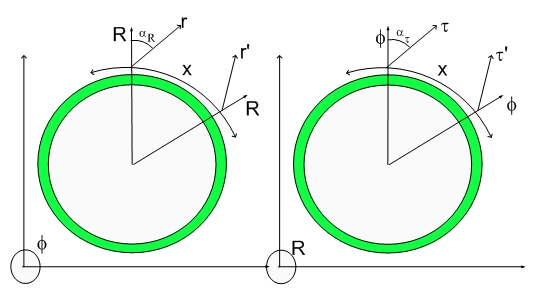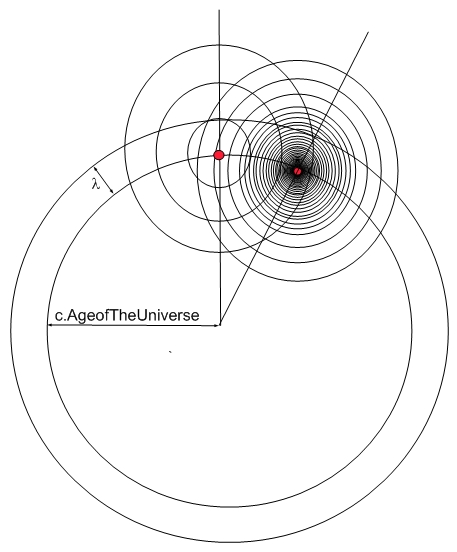# Newton's Third LawNewton's Third Law

By now, I am sure you people are upset that I have been picking on poor Newton. It just happens that his work is fundamental and revising it is a sign of respect and something I couldn't avoid.

The last law states that the force sensed by two interacting bodies are equal and in opposing directions (signs).

This is the easiest one to recast.

I will be terse and brief since the reproduction of the forces is enough to show that they have the same value and opposite signs. I will only give you the physical (geometrical) interpretation of the Quantum Lagrangian Principle.The picture above displays the Universe on the RX cross section (right panel in the Hypergeometrical Universe Icon). The age of the Universe is around 15 billion light years and lambda is the Compton wavelength of an atomic mass unit particle, so this is not draw to the correct scale..:)

I show two bodies about to step one de Broglie wavelength in the Universe lightspeed expansion.

It is impossible to draw things correctly and still be didactic. A large mass body, represented on the right, will have a very small Compton wavelength. In my calculations, I represent their wavelegnth as being zero for simplicity. Under those conditions the single dilator (one a.m.u. mass) interacts with a continuous field (it still oscillates but their maximae are too close together to affect the calculations). I just had to calculate the shift on the next maximae generated by the dilator along the radial direction. In the absence of interaction, the dilator's field would have a maximum exactly along the radial line. In the presence of the interacting body, that maximum shifts sidewise and one just has to calculate a dilaton gradient and equate it to zero (l'Hospital rule..:).

The effect of being a large (macroscopic) body is two-fold: it makes its effective Compton wavelenght to be zero and it scales the intensity by the number of dilators it contains. One kilogram of anything (mass or charge) would contain 1000 Avogrado's number of dilators. Thus the local dilaton field, in the proximity of the large body, would be much larger and the shift would be inversely proportional to that number of dilators.

Since the shift is proportional to the acceleration, one can see that the acceleration in the larger body would be inversely proportional to its "mass".

That should be enough for this law. I am eager to go back to the Hyperons. Please, feel free to ask any questions.

Cheers,

MP

Currently unrated

There are currently no comments

### New Comment

required

required (not published)

optional

required